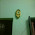Techniques to solve Data Interpretation: Tables problems

Today I would like to discuss a very known topic Data Interpretation of Quantitative Aptitude section. Around 3-4 sets of Data Interpretation are asked in almost every exam. As this is a scoring section, you should practice more to fetch good number of marks.

Steps to solve problems of Data Interpretation: Tables

• Tables are the method of systematic presentation of facts and figures in various rows and columns.
• Before start solving the question, always have a first look of how data is presented.
• Get a proper understanding of presented data.
• Then, start solving the questions using the given data.
• If required, add all the values horizontally and vertically first. Then proceed to questions.

Problems with Solution

Problem1: Study the following table carefully and answer the questions:

 SCHOOLS STANDARDS I II III IV V A 40 55 45 60 45 B 50 60 60 45 55 C 35 50 55 45 50 D 45 55 45 50 45 E 50 50 45 60 50

1. Which school has highest total number of students taking all the standards together?
2. Which standard has lowest total number of students taking all the schools together?

3. What is the ratio of students studying in standard III of schools A and B to those studying in standard V of schools A and B together?

4. What is the average number of students studying in standard I from all schools together?

5. Number of students studying in standard IV of school E is what percentage of students studying in standard IV from school D?

Solution: Firstly, take a minute to read the table and analyze it. After reading the question,You will come to know that you will need the sum of all the rows and columns. So, before start solving the questions, get a sum of all rows and columns.

 SCHOOLS STANDARDS I II III IV V Total A 40 55 45 60 45 245 B 50 60 60 45 55 270 C 35 50 55 45 50 235 D 45 55 45 50 45 240 E 50 50 45 60 50 255 Total 220 270 250 260 245

Now, lets start solving the questions

1. Highest total number of students taking all the standards together
⇒ Maximum value of last column = 270 i.e. School B

2. Lowest total number of students taking all the schools together
⇒Minimum value of last row = 220 i.e. Standard I

3. Standard III of school A and B :  Standard V of school A and B ::  (45+60) : (45+55)
⇒ 105: 95
⇒21: 19

4. Average number of students studying in standard I from all schools together
⇒ (Number of students)/(5) = (220)/5 = 44

5. Let number of students studying in Standard IV from school E = x % of number of students in Standard IV from school D
⇒60 = x% of 50
⇒ x = (60/50) times (100) = 120

Therefore, 150% is the answer.

Problem2: Study the following table carefully to answer the questions

 YEAR STREAM ARTS SCIENCE COMMERCE BOYS GIRLS BOYS GIRLS BOYS GIRLS 2001 500 400 600 500 600 600 2002 700 600 700 600 700 600 2003 600 500 500 500 800 700 2004 800 600 900 900 800 800 2005 700 500 800 800 900 500

1. Number of boys in arts stream in year 2004 is what percentage of total number of boys for all years together in Arts stream?

2. What is the ratio of total number of boys to the total number of girls, taking all the streams together, for year 2005?

3.What is the average number of girls from commerce steam for the given years?

Solution: Study the table first, three streams are mentioned i.e. arts, science and commerce and the students are divided into girls and boys sections.

1.  Number of boys in arts stream in year 2004 = 800

Total number of boys for all years together in Arts stream= 3300

⇒ 800 = x % of 3300

⇒ x = (800)/(3300) times 100

⇒ x= 24.24

2. Total number of boys for year 2005 : Total number of girls for year 2005

⇒700+ 800+900 : 500+ 800+ 500

⇒ 2400: 1800
⇒4:3

3. Average number of girls from commerce steam for the given years
⇒(600+600+700+800+500)/5
⇒(3200)/5
⇒640

I hope you understood the above problems, you are free to ask any query anytime.

What's trending in BankExamsToday

Smart Prep Kit for Banking Exams by Ramandeep Singh - Download here

Join 40,000+ readers and get free notes in your email1.Thank you so much. This is very very helpful.

2.Hi sir
Table 1--------- 5th question answer is wrong pls check it and update with correct answer .

1.thnx dear,,, its 120, not 150,, just a typing mistake...

3.Hello Sir,
Table no 1......3 question addition is wrong pls check it and update with correct answer.

I will try to respond asap